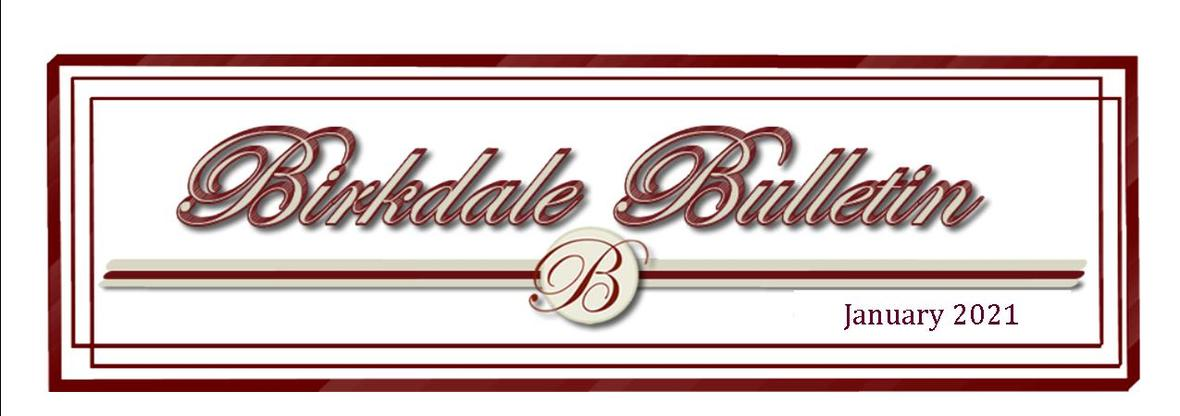## President's Message- January 2021

 /* styles */ We closed out the old year with a couple of events to brighten the holiday mood. On Saturday, December 12th, Santa Claus made a noisy tour of Birkdale. Thanks to all those who made his visit possible and provided the procession through the community. The following evening, judges drove the streets and selected the winners of our decorations contest. Congratulations to Justin & Rachel Clouse and family at 14515 Leamington Drive, our Grand Prize winners. See a complete story on the many homes considered elsewhere in this newsletter. And now we are kicking off another New Year, 2021. What will this New Year hold for us? Other than those proverbial standbys, death and taxes, it is hard to predict. We can likely anticipate among our families or friends that there will be some marriages, some births, some illnesses, some celebrations, and some heartaches. But, whatever comes, we can anticipate some surprises and some expected events. We would have to have been hermits not to notice the work taking place along Winterpock Road and Spring Run Road. These activities are the result of the growth in residential communities surrounding Birkdale. And we are not immune to some of those necessary improvements. Recently I met with representatives of Dominion Energy to review impending expansion of three power substations in this part of Chesterfield County. Work on “our” substation behind the green on Hole 14—to the west of Turning Lane—should begin sometime this summer. Access to the site will be from Winterpock Road, which should not affect Birkdale, but there will be some tree removal and construction activity for about six months. Lastly, the BCA board continues to work through some issues left over from the previous meetings. The most serious of these matters is nomination of a fifth board member to complete our membership. We have discussed several individuals and discussed an appointment with others, but we continue to seek qualified and interested persons for this position. We welcome recommendations from residents. As we start this year, let’s strive to be what one insurance company has set as its goal, let’s all try to be “good neighbors.” Ron Buchanan President, Birkdale Community Association
 table div table+table+table+table+table div table{width:100%;padding:0}table div table+table+table+table+table div table img{width:96.23%;padding:0;float:none}table div table+table+table+table+table div table td{width:100%;padding:0 1.88% 18px}/* styles */# Next Board of Directors Meeting

The next Birkdale Board of Directors Meeting will take place

Feburary 4th, 2021

6 pm at the Birkdale Club House.

All residents are encouraged to attend.

 table div table+table+table+table+table+table+table div table{width:100%;padding:0}table div table+table+table+table+table+table+table div table img{width:96.23%;padding:0;float:none}table div table+table+table+table+table+table+table div table td{width:100%;padding:0 1.88% 18px}/* styles */## Treasurer's Report- January

 table div table+table+table+table+table+table+table+table+table+table+table div table{width:100%;padding:0}table div table+table+table+table+table+table+table+table+table+table+table div table img{width:96.23%;padding:0;float:none}table div table+table+table+table+table+table+table+table+table+table+table div table td{width:100%;padding:0 1.88% 18px}/* styles */# Birkdale's Most Recent Financial Statement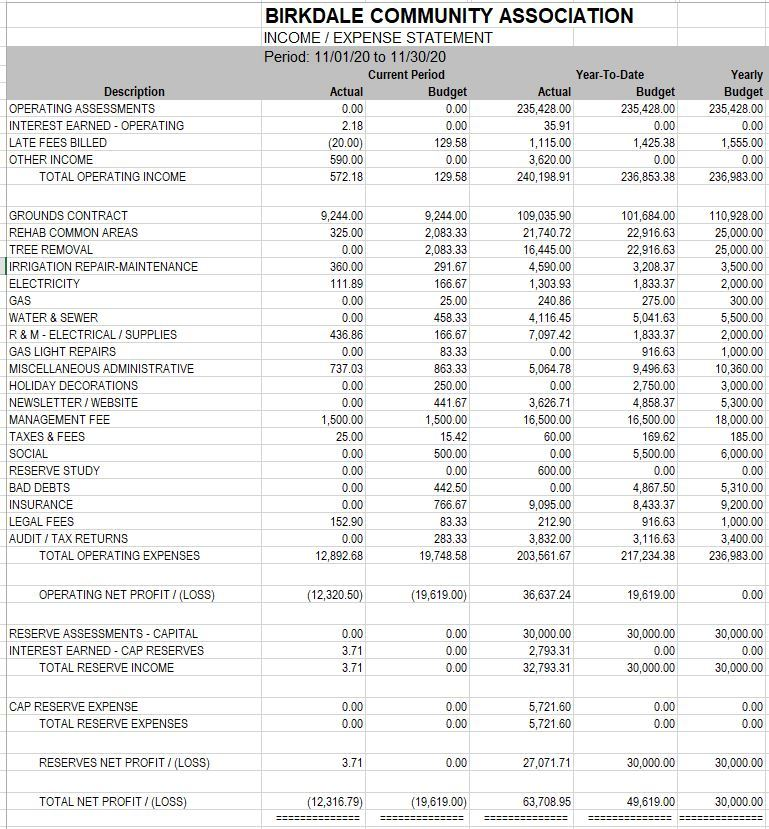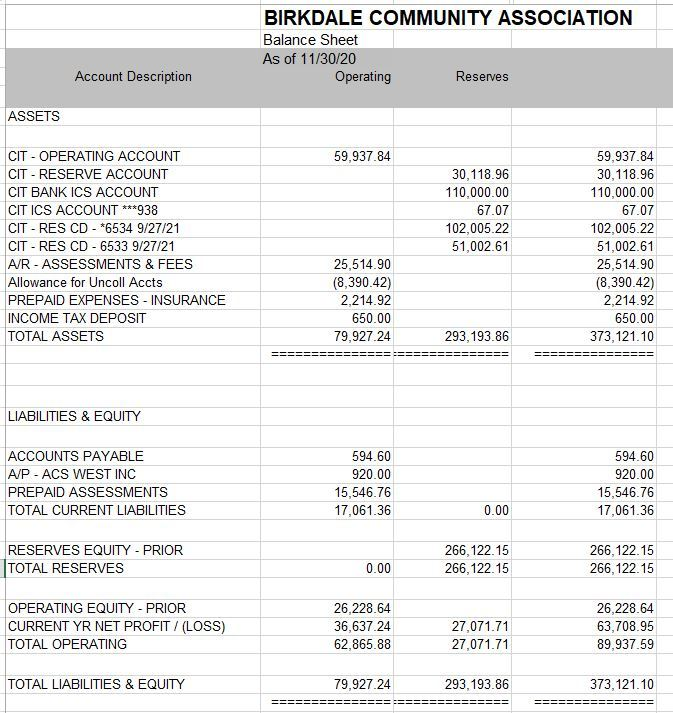table div table+table+table+table+table+table+table+table+table+table+table+table+table+table+table div table{width:100%;padding:0}table div table+table+table+table+table+table+table+table+table+table+table+table+table+table+table div table img{width:96.23%;padding:0;float:none}table div table+table+table+table+table+table+table+table+table+table+table+table+table+table+table div table td{width:100%;padding:0 1.88% 18px}/* styles */# ACS West is our Managing Agent

You may use your coupon book to send in your payment or set up quarterly payments through ACS West or your bank.

REMINDER: Assessments were due January 1st.

Thank you!

 table div table+table+table+table+table+table+table+table+table+table+table+table+table+table+table+table+table div table{width:100%;padding:0}table div table+table+table+table+table+table+table+table+table+table+table+table+table+table+table+table+table div table img{width:96.23%;padding:0;float:none}table div table+table+table+table+table+table+table+table+table+table+table+table+table+table+table+table+table div table td{width:100%;padding:0 1.88% 18px}/* styles */## News from the Architectural Review Committee“﻿Welcome to 2021.” We all are hoping this year will be an improvement over the pandemic year of 2020. Please be safe and follow the health requirements that are in place throughout the community.
Just think spring ... is just around the corner. This is when the community can continue to work on their home projects to beautify the neighborhood.

HAPPY NEW YEAR from the ARC committee!

Applications submitted to ARC for approval:

8307 Houghton Place - Playscape
9049 Spyglass Hill Turn - Repair walkway
9325 Mission Hill Lane - Playscape

Happy Holidays from the ARC.

Lee Kemmet
ARC Committee

## Mailbox Maintenance

Mailbox maintenance is the homeowner’s responsibility, and the specific requirements are in the ARC Guidelines.

When painting your mailbox, Lowe’s on Hull Street has the equivalent paint color as Benjamin Moore which is Lowe's - Valspar, Quart, 372, Birkdale Vanilla Cookie, Exterior Satin, Duramax, 105-1, 107-1, 214-2.5.

For your metal mailbox, Cottage Red Benjamin Moore is available at Virginia Paint and can also be mixed at Lowes.

The Birkdale logo and numbers may be purchased from Sign Crafters Inc., Attn: Barbara Isenberg, 804-379-2004 or email bisenberg@sign-crafters.com

OR

You may contact resident Rich McGinness - The Mailbox Guy- who will assist you with mailbox maintenance.

Rich McGuinness at Themailboxguy@yahoo.com or call 919-621-9983

 table div table+table+table+table+table+table+table+table+table+table+table+table+table+table+table+table+table+table+table+table div table{width:100%;padding:0}table div table+table+table+table+table+table+table+table+table+table+table+table+table+table+table+table+table+table+table+table div table img{width:96.23%;padding:0;float:none}table div table+table+table+table+table+table+table+table+table+table+table+table+table+table+table+table+table+table+table+table div table td{width:100%;padding:0 1.88% 18px}/* styles */## Thank You to all the Kind Neighbors and Those who Volunteered Their Time in 2020. We Appreciate All of You!

 table div table+table+table+table+table+table+table+table+table+table+table+table+table+table+table+table+table+table+table+table+table+table+table+table div table{width:100%;padding:0}table div table+table+table+table+table+table+table+table+table+table+table+table+table+table+table+table+table+table+table+table+table+table+table+table div table img{width:96.23%;padding:0;float:none}table div table+table+table+table+table+table+table+table+table+table+table+table+table+table+table+table+table+table+table+table+table+table+table+table div table td{width:100%;padding:0 1.88% 18px}/* styles */2021 BIRKDALE PROPOSED COMMUNITY ASSOCIATION CALENDAR

*The calendar will be finalized at the first 2021 Board Meeting

February 4th- Board Meeting

May 6th - Board Meeting

May 8th- Semi-Annual Spring Yard Sale

August 5th- Board Meeting

September 9th-Board Budget Meeting

October 2nd- Semi-Annual Fall Yard Sale

October 7th- Annual Board Meeting

October 16th- Fall Festival

November 6th- Board Meeting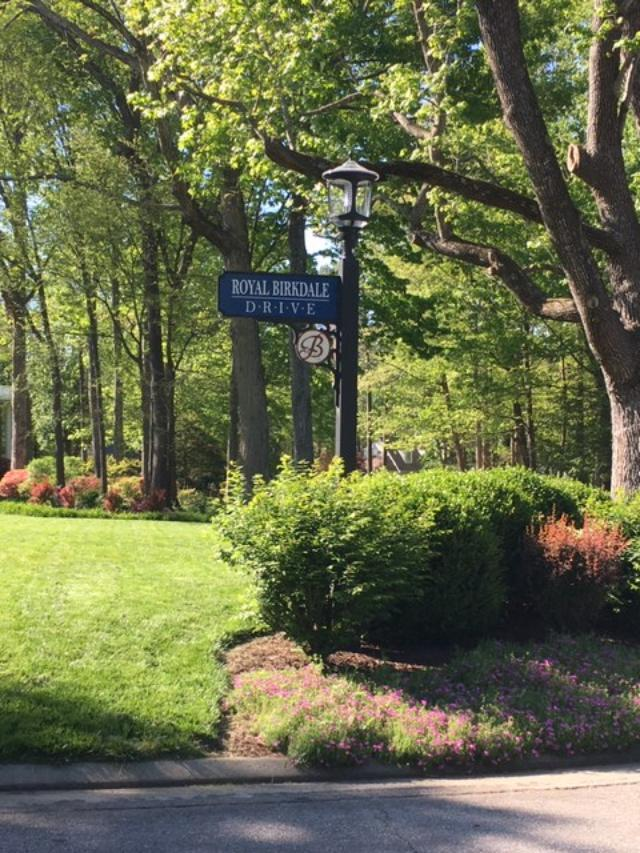# Landscape & Neighborhood Etiquette

NO DUMPING- Please do not drag brush and clippings or debris into the common areas to include the area surrounding the power lines behind Royal Birkdale. Those areas belong to you and to me as property owners.

PARKING- Do not park on the green spaces in Birkdale. Please park in your driveway rather than on the street fronting your home. Street parking limits access to emergency vehicles, causes congestion, and is a hazard for children and walkers.

NO FIRES whatsoever. Fires require a permit from Chesterfield County. It is not appropriate to burn any vegetation in common areas and cause alarm to nearby homeowners.

TRASH- Birkdale has a backyard trash policy. Please do not take you trash cans to the curb. There are many companies who offer backyard pickup services in our neighborhood.

DOG WASTE- Please be courteous and pick up after your pooch. While walking please make an effort to keep your dog from using the bathroom on other resident's property. Direct them to the mulched common areas whenever possible-and pick up!

If you have any problems or complaints regarding common areas, please call Wayne Bass at 804-639-0795.

# Lighting

Gas street lights were replaced with solar powered LED lights last year and are now the standard from the Winterpock Road entrance to the Spring Run Road entrance. Solar power is dependent on adequate sunlight to recharge the batteries. Thus in winter, lights may not function all night. If you have questions or notice a situation which may need attention, call ACS West for investigation.

 table div table+table+table+table+table+table+table+table+table+table+table+table+table+table+table+table+table+table+table+table+table+table+table+table+table+table+table div table{width:100%;padding:0}table div table+table+table+table+table+table+table+table+table+table+table+table+table+table+table+table+table+table+table+table+table+table+table+table+table+table+table div table img{width:96.23%;padding:0;float:none}table div table+table+table+table+table+table+table+table+table+table+table+table+table+table+table+table+table+table+table+table+table+table+table+table+table+table+table div table td{width:100%;padding:0 1.88% 18px}/* styles */# Covenants Committee

JANUARY 2021

The Covenants Committee thanks all the residents for your cooperation in addressing Architectural Standards violations or variances. Adherence to these standards improves the overall property quality in Birkdale and benefits us all by maintaining or improving property values and neighborhood quality. The year ends with a few outstanding cases which will be addressed in the new year. There also were several cases from the annual review which will need to be addressed in 2021.
The Committee would also like to remind residents of a few recurring items which, if attended to, will reduce the number of violation notices.

Trash cans – Birkdale Declarations and ARC Standards established Birkdale as a community in which trash cans are to be stored behind the house of in a screened area. Curbside trash collection is not allowed. New residents frequently are not aware of this.

Landscaping maintenance – Shrubbery shall consist of a minimum standard of plants which should be maintained, trimmed, or pruned as needed. Lawns should be mowed to an appropriate level but must be below 5 inches height. Trees in Birkdale are showing more deterioration due to age. Dead trees should be removed and replacing of trees should be considered.

Power washing - Houses should be kept clean from mildew or other material which soils it. This is especially common on the north side of houses.

Mailboxes – Mailbox posts and the mailboxes are the most frequent source of violations. Proper maintenance will allow these to last a long time. Posts and mailboxes should be painted the correct colors (Vanilla Cookie and Cottage Red) as specified in the ARC Standards. Mailbox posts are especially vulnerable to water damage at the top which is an open grain of the wood. These can be preserved by use of sealing products, such as KILZ or wood filler, prior to painting.
The media box and accent pieces may be constructed with PVC rather than pine to extend the life.

Holiday Decorations – ARC Guidelines 6.21 D addresses the installation and removed of holiday lighting. ARC approval is not required for installation of holiday or festive lighting. Lighting must coincide with the holiday and must be removed within 14 days of the holiday, i.e. January 7th. **

 table div table+table+table+table+table+table+table+table+table+table+table+table+table+table+table+table+table+table+table+table+table+table+table+table+table+table+table+table+table div table{width:100%;padding:0}table div table+table+table+table+table+table+table+table+table+table+table+table+table+table+table+table+table+table+table+table+table+table+table+table+table+table+table+table+table div table img{width:96.23%;padding:0;float:none}table div table+table+table+table+table+table+table+table+table+table+table+table+table+table+table+table+table+table+table+table+table+table+table+table+table+table+table+table+table div table td{width:100%;padding:0 1.88% 18px}/* styles */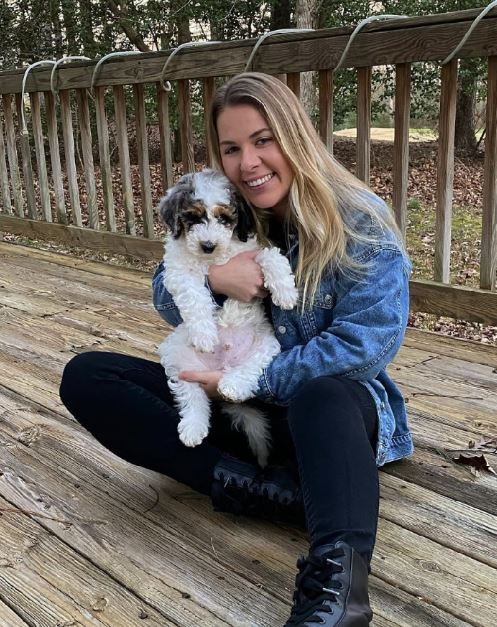# Birkdale's Pet of the Month

Meet Zeke-an adorable Aussie Doodle pup recently adopted by Hanna Fitzgerald. Congratulations on your new fur baby!

Would you like to see your pet featured in the Bulletin?

Send us a photo of your pet and a brief description to appear as next month's Pet of the Month

email to:
Subject line- Pet of the Month

 table div table+table+table+table+table+table+table+table+table+table+table+table+table+table+table+table+table+table+table+table+table+table+table+table+table+table+table+table+table+table+table div table{width:100%;padding:0}table div table+table+table+table+table+table+table+table+table+table+table+table+table+table+table+table+table+table+table+table+table+table+table+table+table+table+table+table+table+table+table div table img{width:96.23%;padding:0;float:none}table div table+table+table+table+table+table+table+table+table+table+table+table+table+table+table+table+table+table+table+table+table+table+table+table+table+table+table+table+table+table+table div table td{width:100%;padding:0 1.88% 18px}/* styles */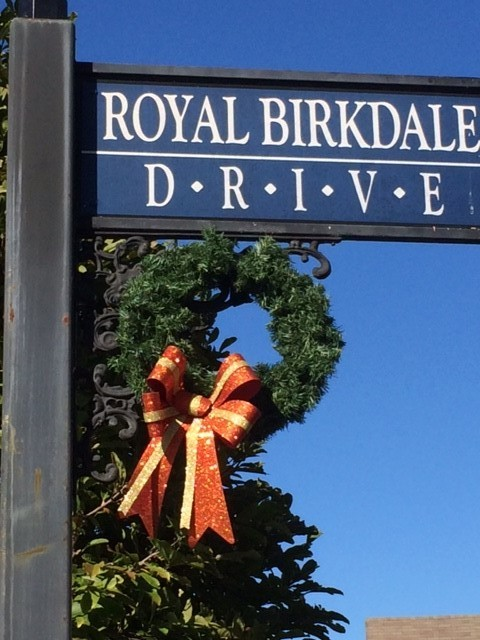# THANK YOU TO OUR HOLIDAY DECORATORS-

This year we would like to thank the following "2020 Wreath Volunteers" for coming out and donating their time to make Birkdale look festive for the holidays.

Baugh, Bishop, Calhoun, Haring, Lasorsa, Lackey
Lowe, Maxwell, Moore, Nevel, Oliver, Sugumele
Wallace

A Special Thank You to Betsy and Nick Martello for their many year of volunteering with organizing the hanging of the wreaths.

Christine Nevel
Csquared122@verizon.net
804-852-8949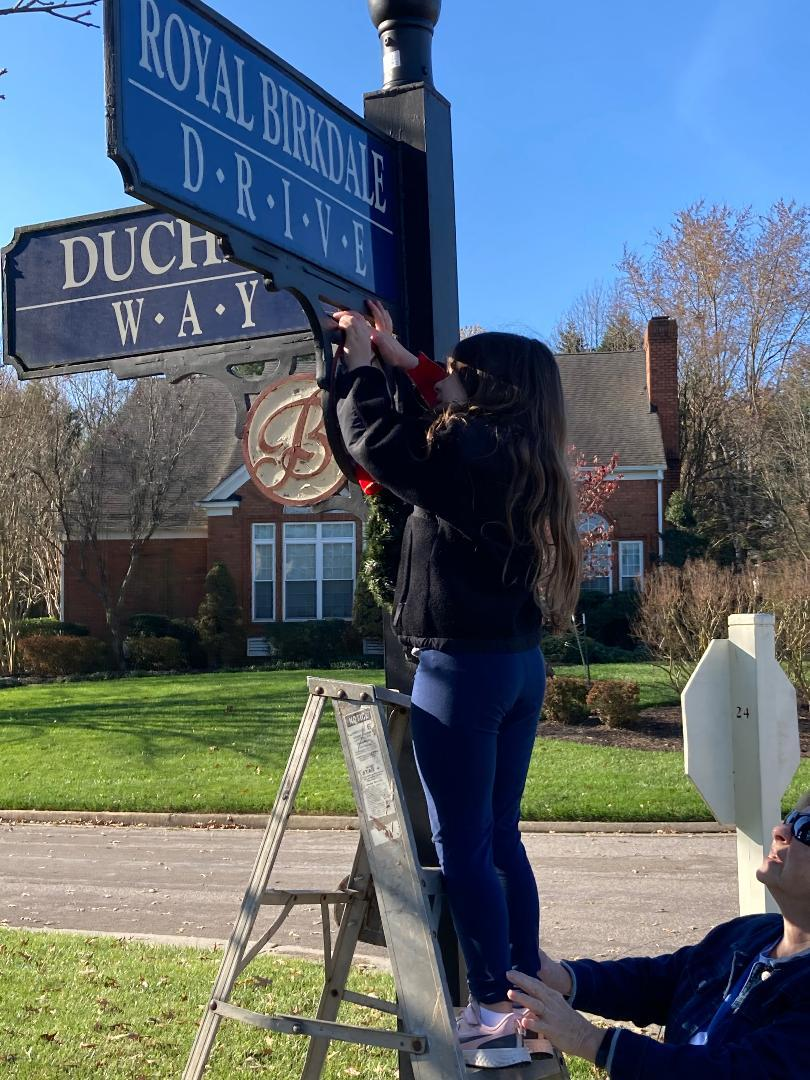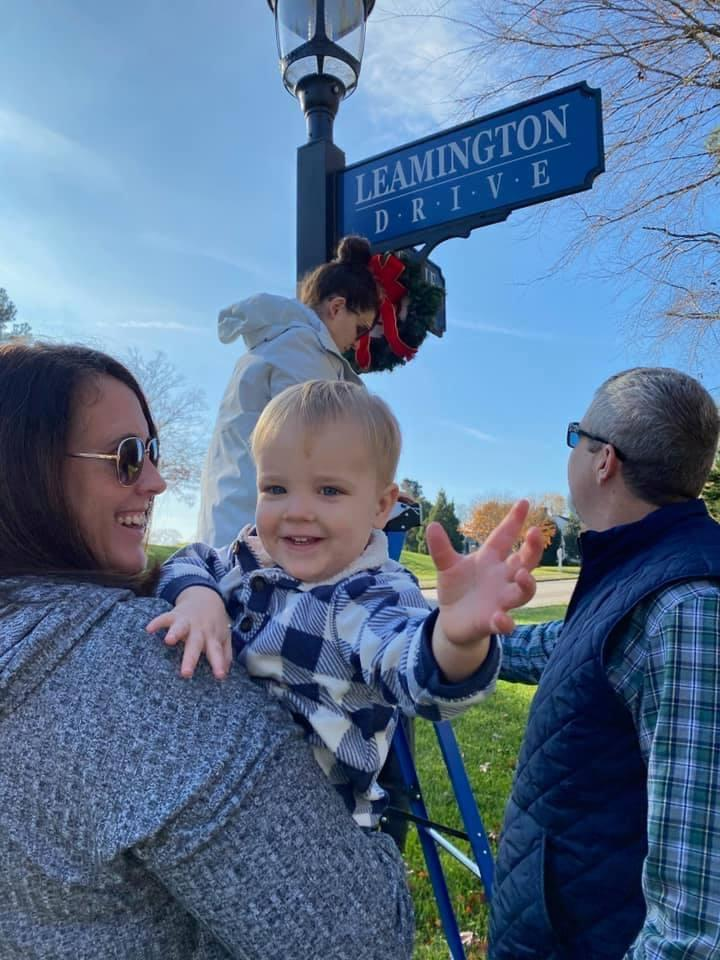table div table+table+table+table+table+table+table+table+table+table+table+table+table+table+table+table+table+table+table+table+table+table+table+table+table+table+table+table+table+table+table+table+table+table div table{width:100%;padding:0}table div table+table+table+table+table+table+table+table+table+table+table+table+table+table+table+table+table+table+table+table+table+table+table+table+table+table+table+table+table+table+table+table+table+table div table img{width:96.23%;padding:0;float:none}table div table+table+table+table+table+table+table+table+table+table+table+table+table+table+table+table+table+table+table+table+table+table+table+table+table+table+table+table+table+table+table+table+table+table div table td{width:100%;padding:0 1.88% 18px}/* styles */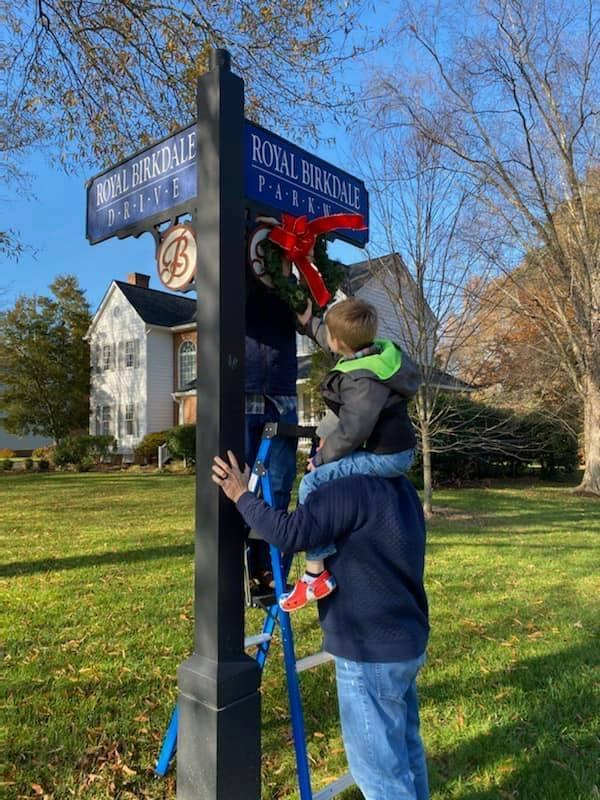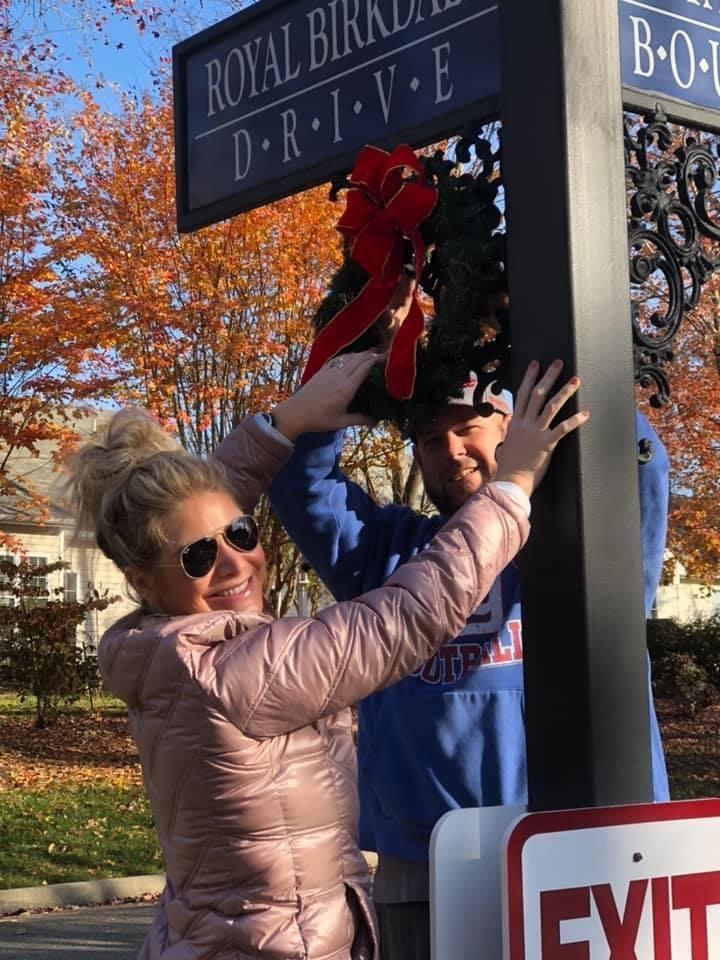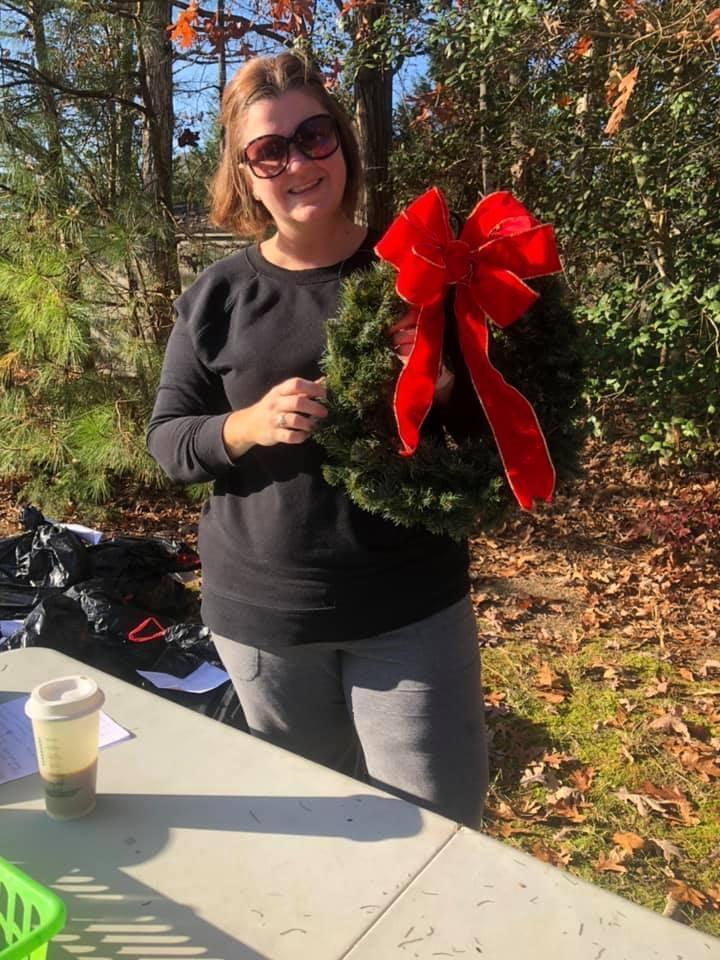table div table+table+table+table+table+table+table+table+table+table+table+table+table+table+table+table+table+table+table+table+table+table+table+table+table+table+table+table+table+table+table+table+table+table+table+table div table{width:100%;padding:0}table div table+table+table+table+table+table+table+table+table+table+table+table+table+table+table+table+table+table+table+table+table+table+table+table+table+table+table+table+table+table+table+table+table+table+table+table div table img{width:96.23%;padding:0;float:none}table div table+table+table+table+table+table+table+table+table+table+table+table+table+table+table+table+table+table+table+table+table+table+table+table+table+table+table+table+table+table+table+table+table+table+table+table div table td{width:100%;padding:0 1.88% 18px}/* styles */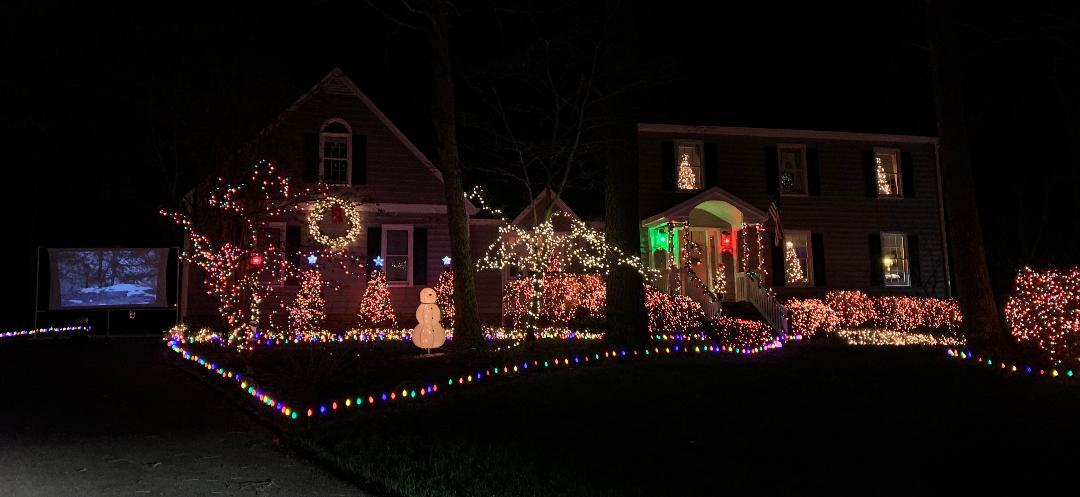# 2020 Outdoor House Decorating Contest

During the truly difficult 2020 year, our neighbors really jumped in with spirit, a sense of fun and wonder, and a bit of competition to bring cheer to us all during the holiday season! The bright sparkling lights, the gigantic inflatables, the smiling and waving Santa Clauses, and remembrances of the true meaning of Christmas were shining bright. We want to thank all of our neighbors for brightening our spirits! Our volunteer judges viewed every house in Birkdale on December 13th to bring back a list of their favorites. The Grand Prize winner was Justin & Rachel Clouse and family at 14515 Leamington Drive, where their house featured a full screen “Elf Movie”, music, inflatables, lights galore and a festive tree in every window! They received a \$50 Lowe’s gift card and a yard sign announcing them as the 2020 winners. The two runner up winners each received a \$25 Lowe’s gift card. So, the winners’ list was:

## Winners Grand Prize

14515 Leamington Drive

Runner Up 8613 Royal Birkdale Drive

Runner Up 8924 Sawgrass Place

## Honorable Mentions

Inflatables & Kids delight Classic Christmas

8907 Royal Birkdale Drive 8848 Torrey Pines Drive

8578 Sunningdale Terrace 8102 Turning Lane

8555 Easton Ridge Place 14514 Leamington Drive

14413 Mission Hills Loop 8807 Merseyside Lane

8022 Ainsdale Lane

8904 Highgate Hill

Lights Galore Homespun Christmas

8543 Sunningdale Terrace 14601 Spyglass Hill Circle

9325 Mission Hills Lane

9015 Spyglass Hill Mews

9142 Stonecreek Club Place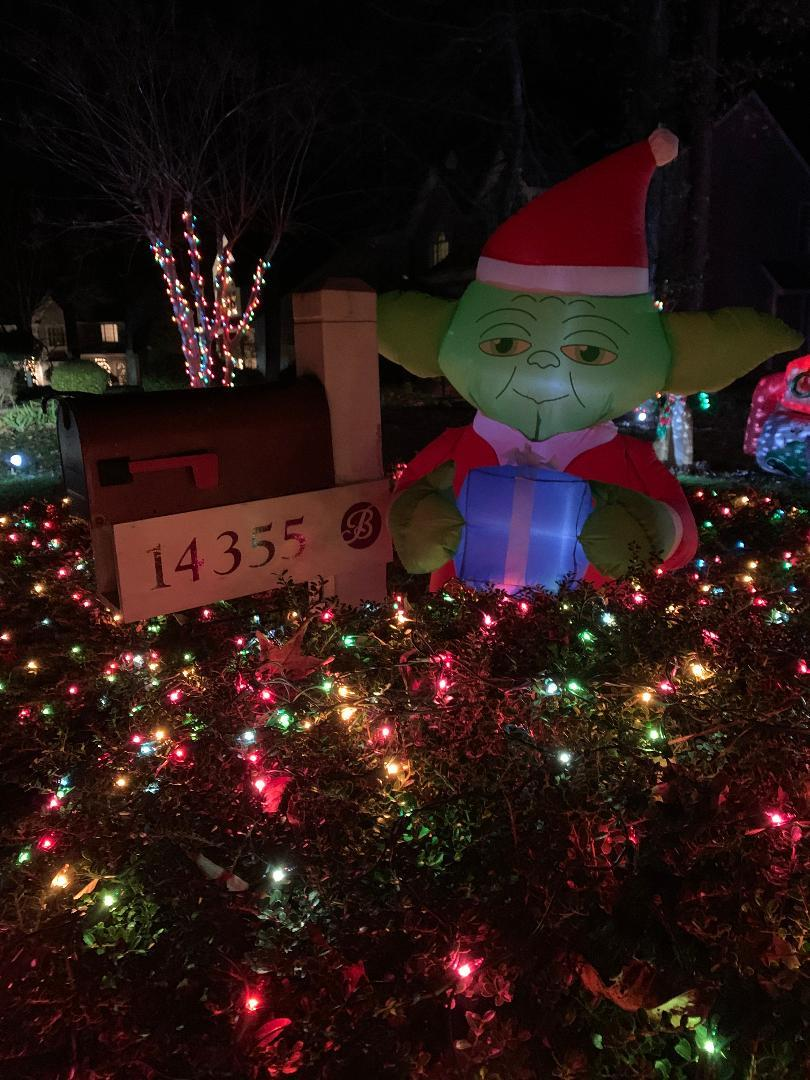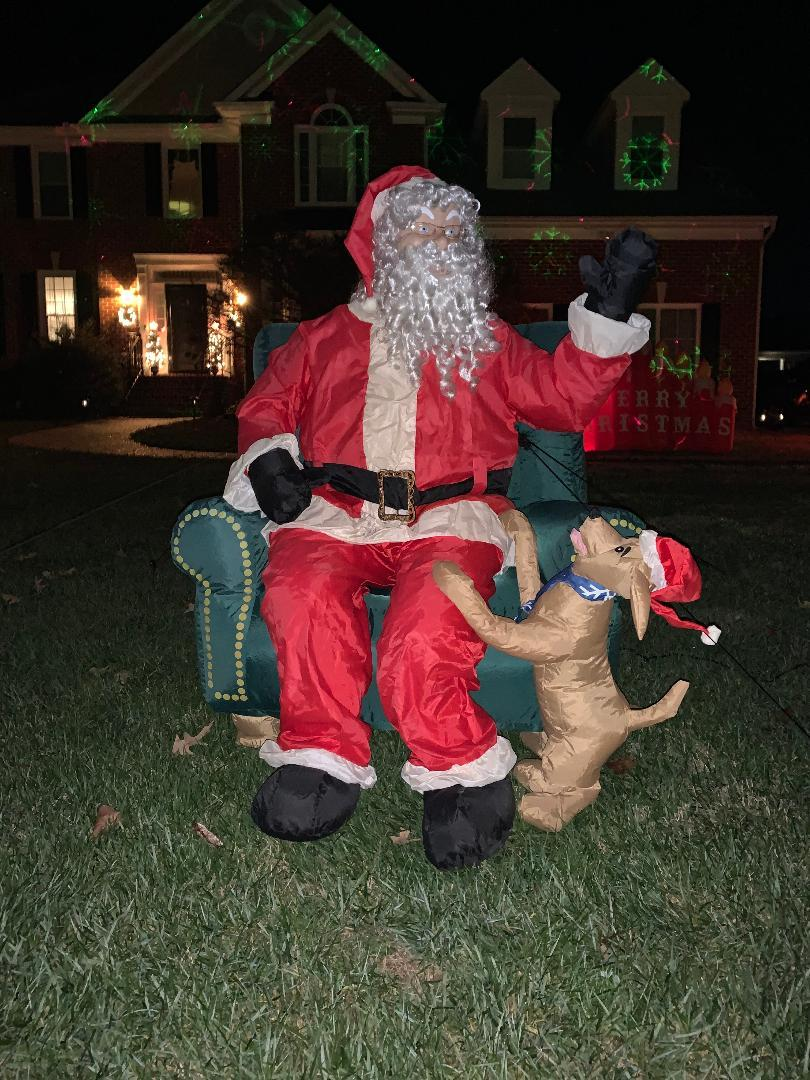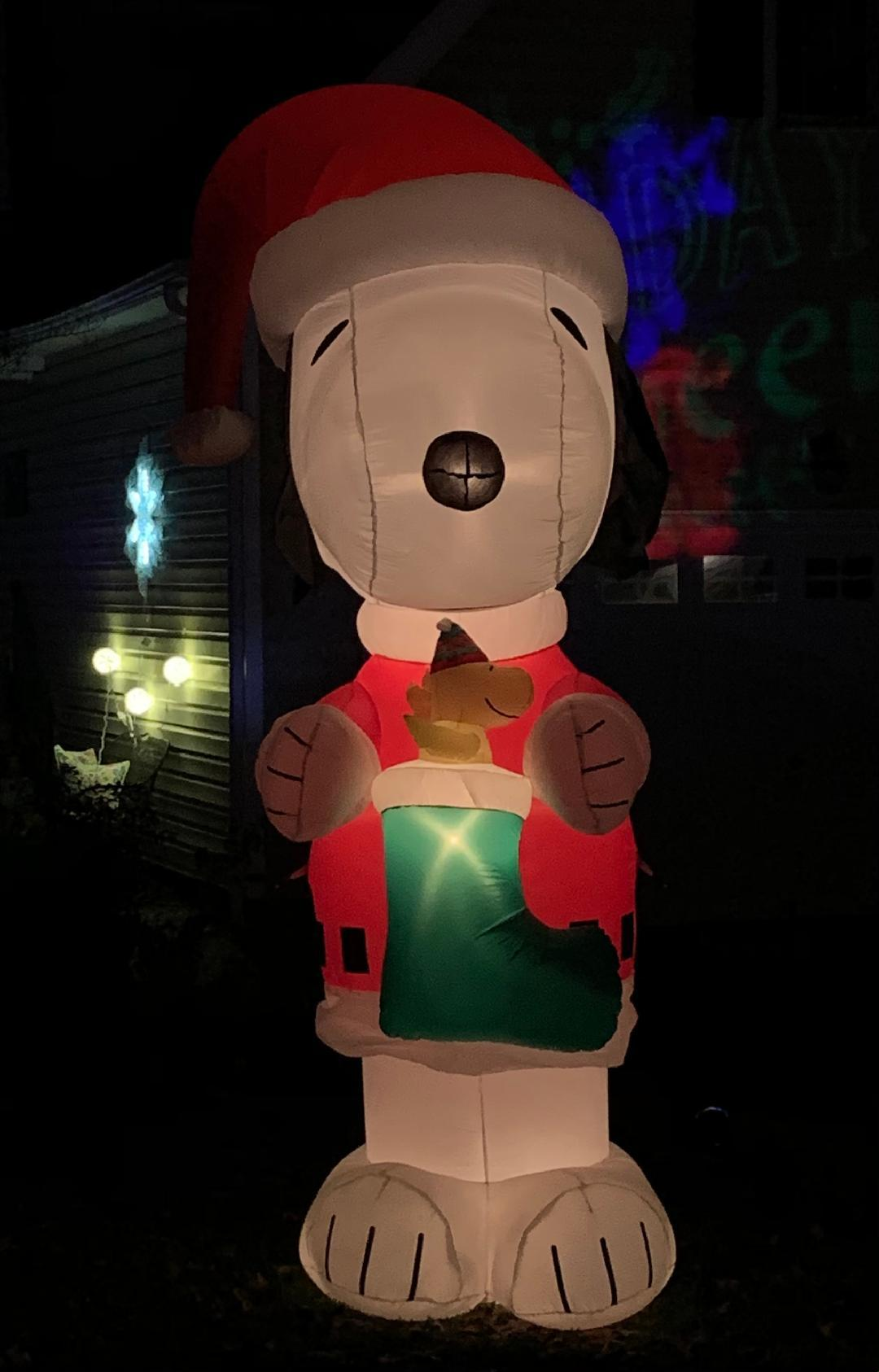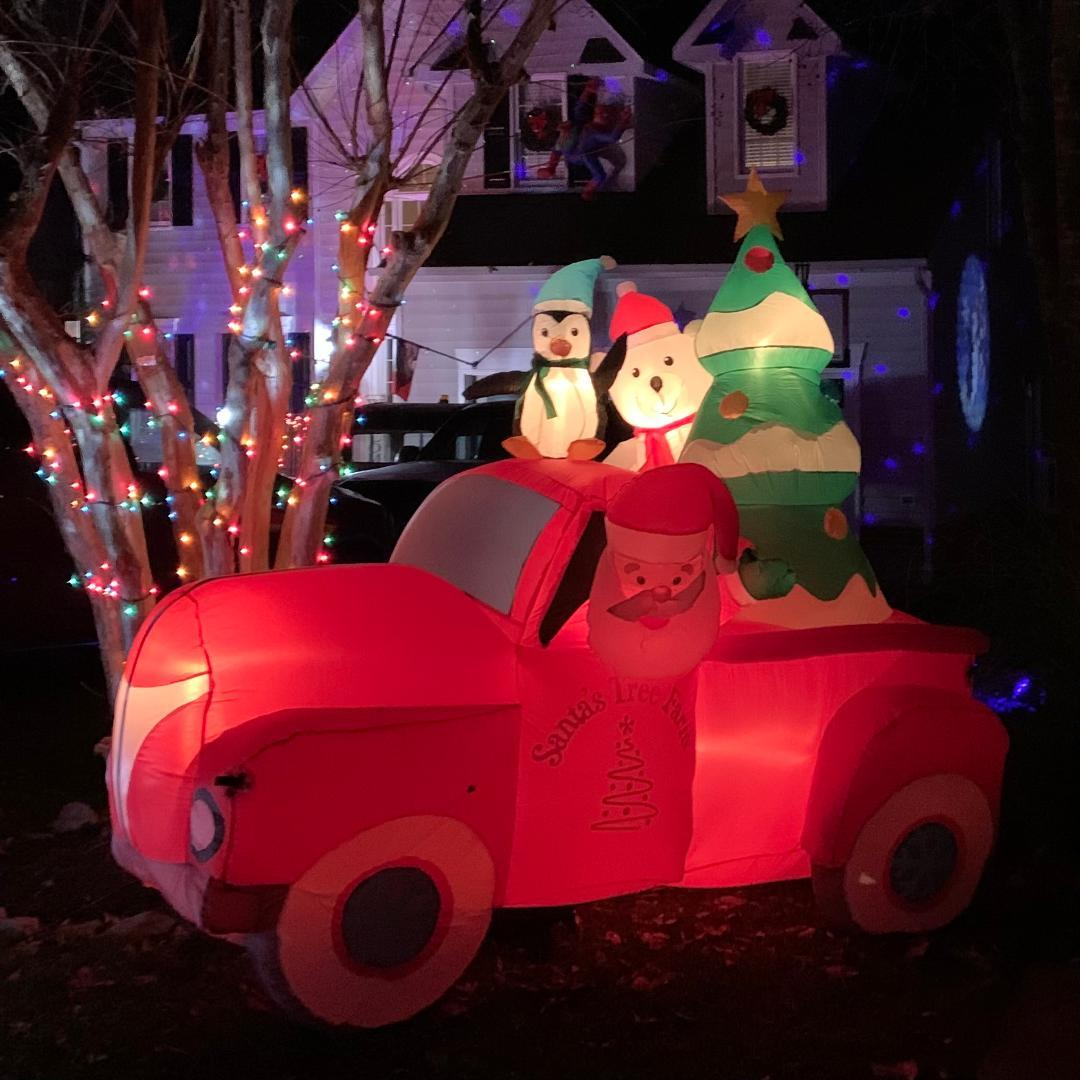table div table+table+table+table+table+table+table+table+table+table+table+table+table+table+table+table+table+table+table+table+table+table+table+table+table+table+table+table+table+table+table+table+table+table+table+table+table+table+table+table+table div table{width:100%;padding:0}table div table+table+table+table+table+table+table+table+table+table+table+table+table+table+table+table+table+table+table+table+table+table+table+table+table+table+table+table+table+table+table+table+table+table+table+table+table+table+table+table+table div table img{width:96.23%;padding:0;float:none}table div table+table+table+table+table+table+table+table+table+table+table+table+table+table+table+table+table+table+table+table+table+table+table+table+table+table+table+table+table+table+table+table+table+table+table+table+table+table+table+table+table div table td{width:100%;padding:0 1.88% 18px}/* styles */# Santa Drive By

Santa’s sleigh visited Birkdale on Saturday, December 12th, with a “ho ho ho” and “Merry Christmas”! Elves led the way in an elf car decorated up top with an elf jack-in-the-box and tossing treats for all! For many families this was the only opportunity to get that quintessential 2020 Santa picture with the kids! But actually, kids of all ages laughed, waved, clapped, grabbed candy and shared the joyful, exuberant event. We want to thank the volunteers that made it possible including Earl Bishop, Nick Wennberg, John Murphy, Anne Haring, Jason Lipp and Marissa Lipp.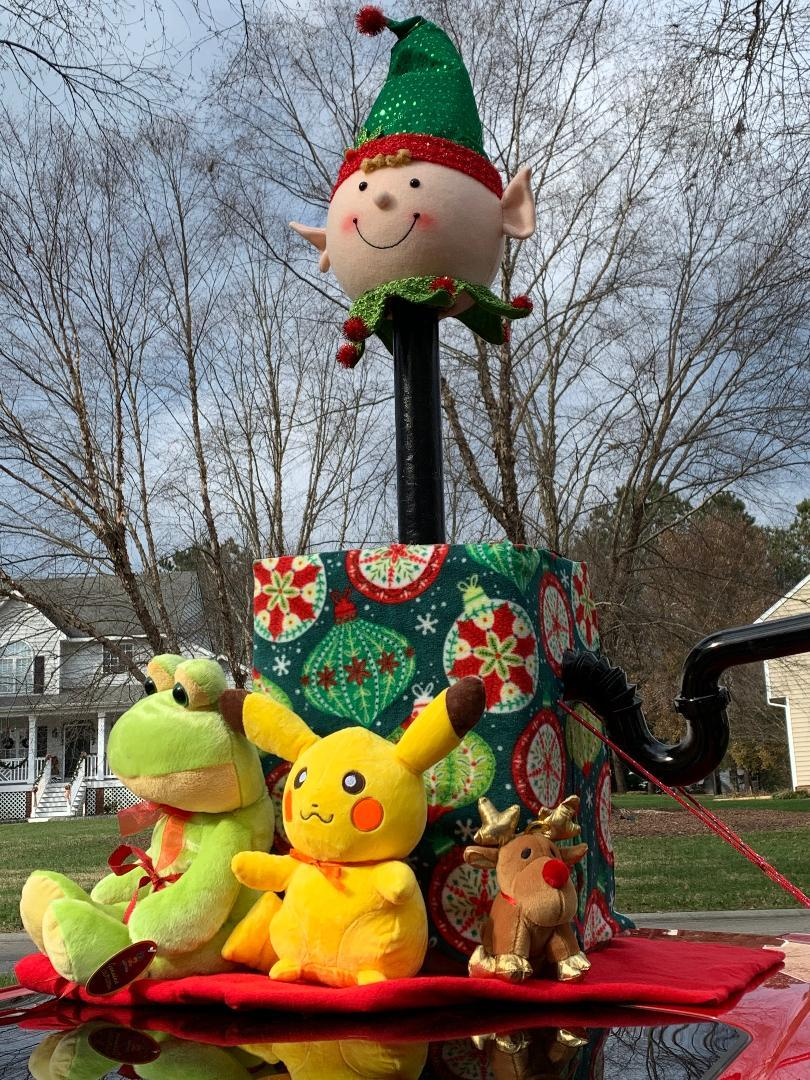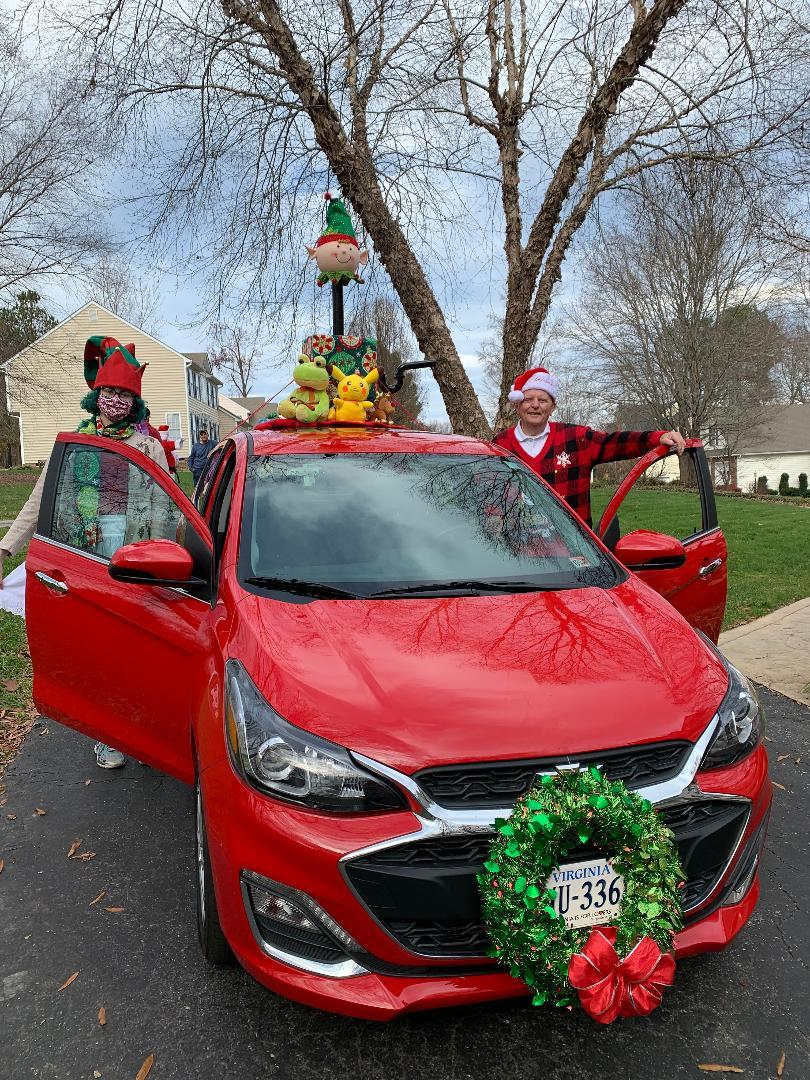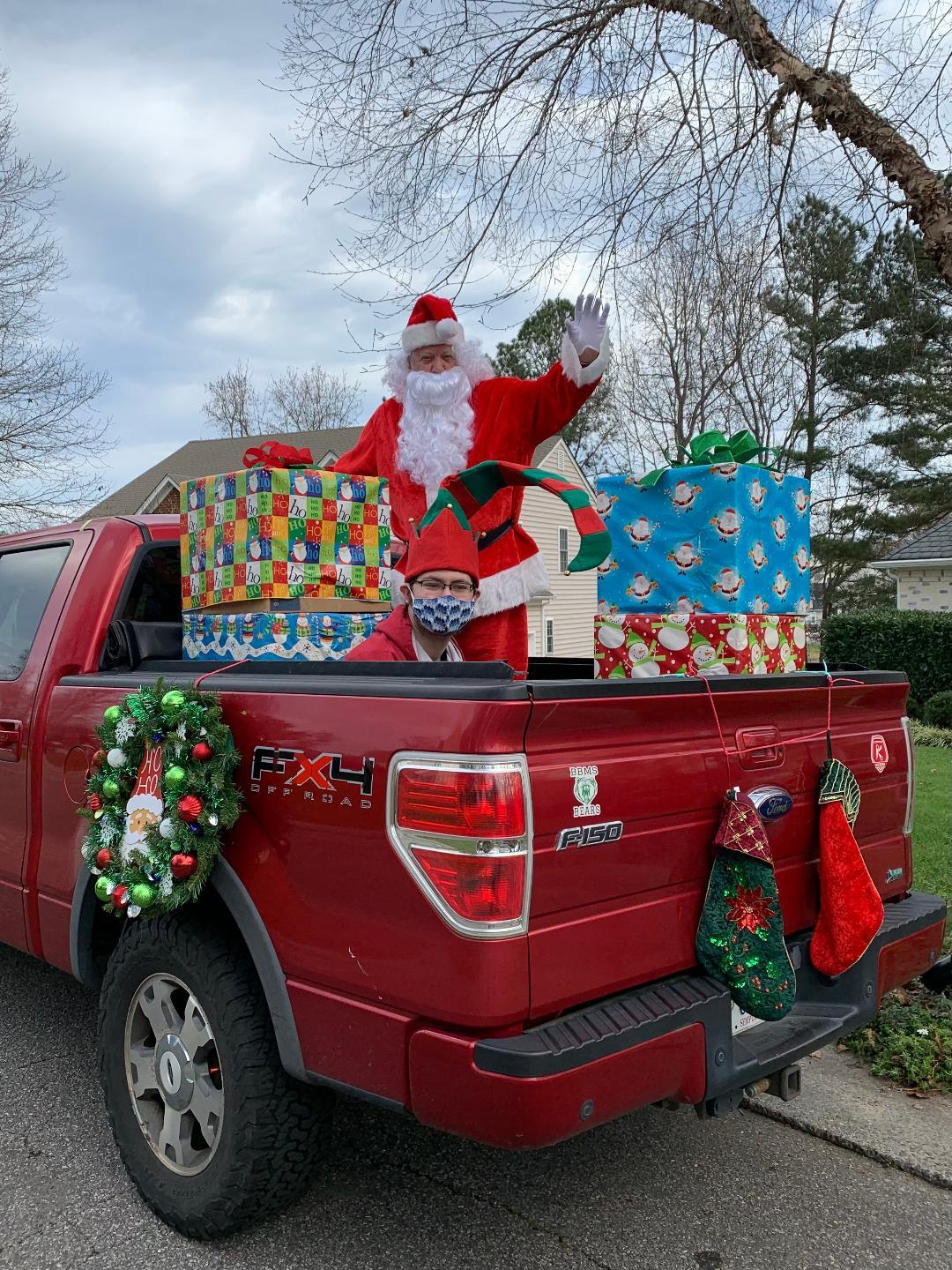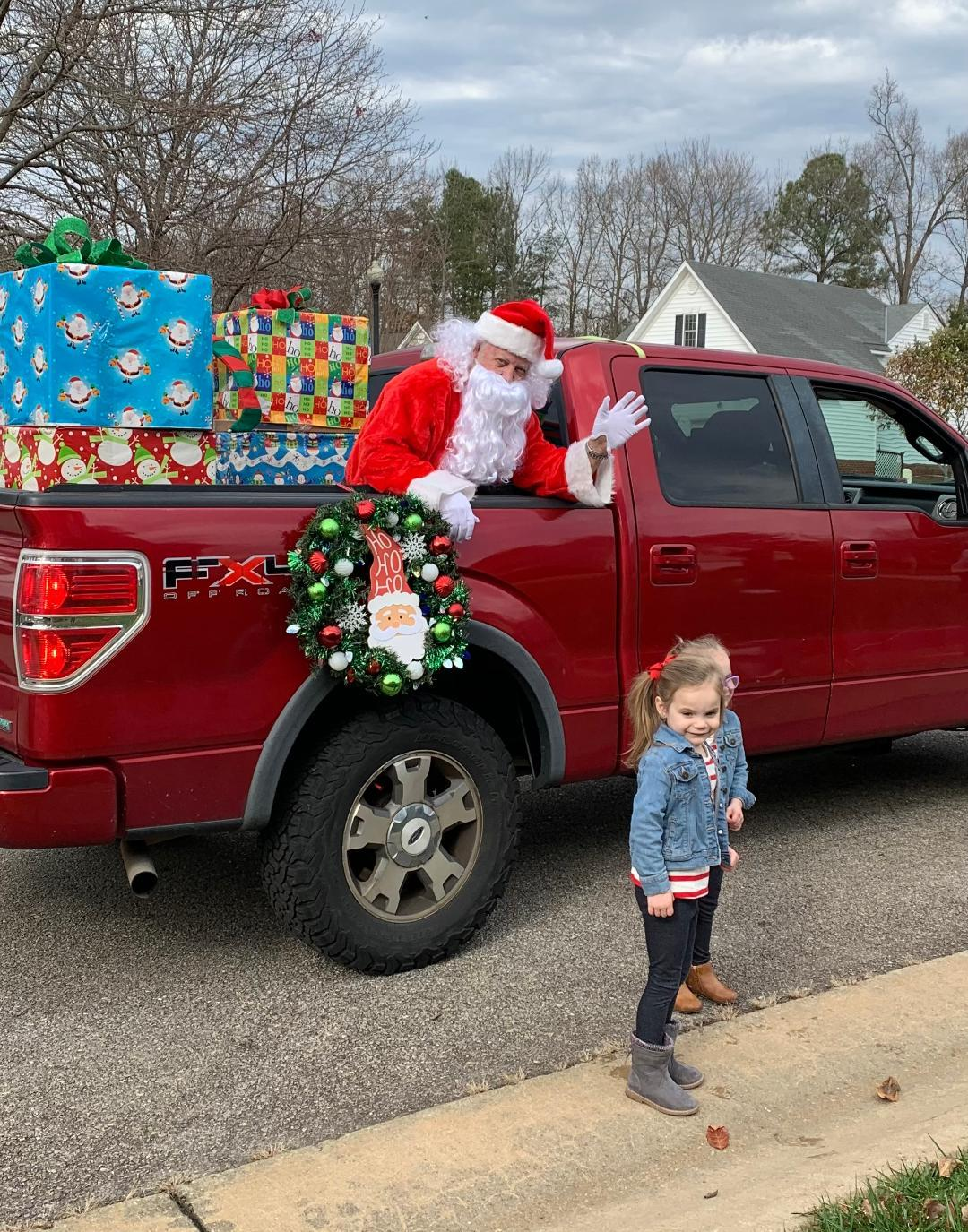table div table+table+table+table+table+table+table+table+table+table+table+table+table+table+table+table+table+table+table+table+table+table+table+table+table+table+table+table+table+table+table+table+table+table+table+table+table+table+table+table+table+table+table+table+table div table{width:100%;padding:0}table div table+table+table+table+table+table+table+table+table+table+table+table+table+table+table+table+table+table+table+table+table+table+table+table+table+table+table+table+table+table+table+table+table+table+table+table+table+table+table+table+table+table+table+table+table div table img{width:96.23%;padding:0;float:none}table div table+table+table+table+table+table+table+table+table+table+table+table+table+table+table+table+table+table+table+table+table+table+table+table+table+table+table+table+table+table+table+table+table+table+table+table+table+table+table+table+table+table+table+table+table div table td{width:100%;padding:0 1.88% 18px}/* styles */# Chesterfield Students Resume an all Virtual Schedule Through the end of January.

With Covid cases exceeding 25 in 100,000 cases, Chesterfield County Schools resuming virtual classes. For information on schedules and updates please visit the county website at https://mychesterfieldschools.com/

Please continue to slow down when driving through the neighborhood as children will be out riding bikes and playing. Please avoid parking in the streets as it blocks the view and affects the safety of everyone.

Winter Break is December 21st-January 1st. Here is the full 2020-2021 School Calendar.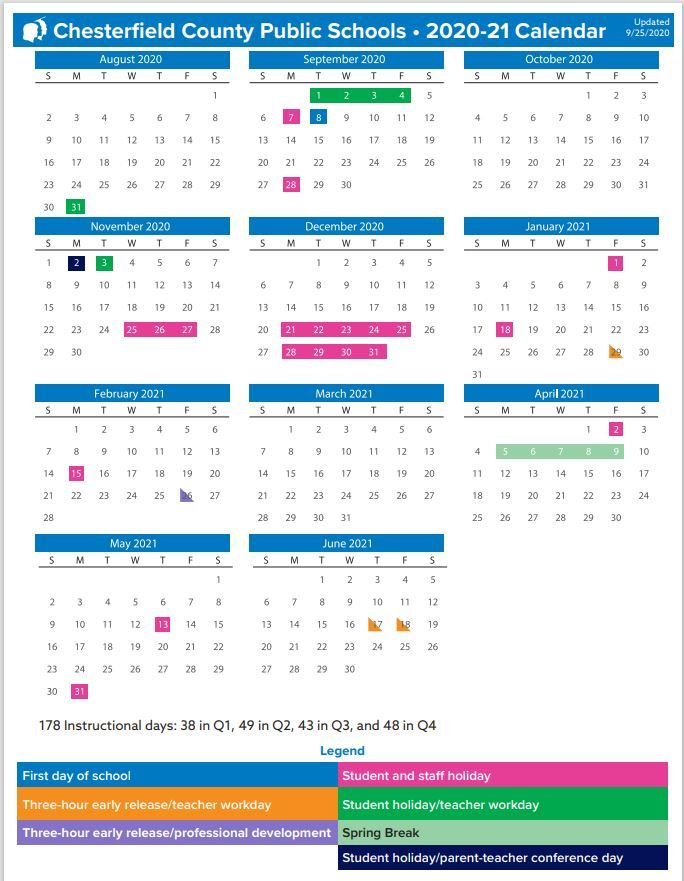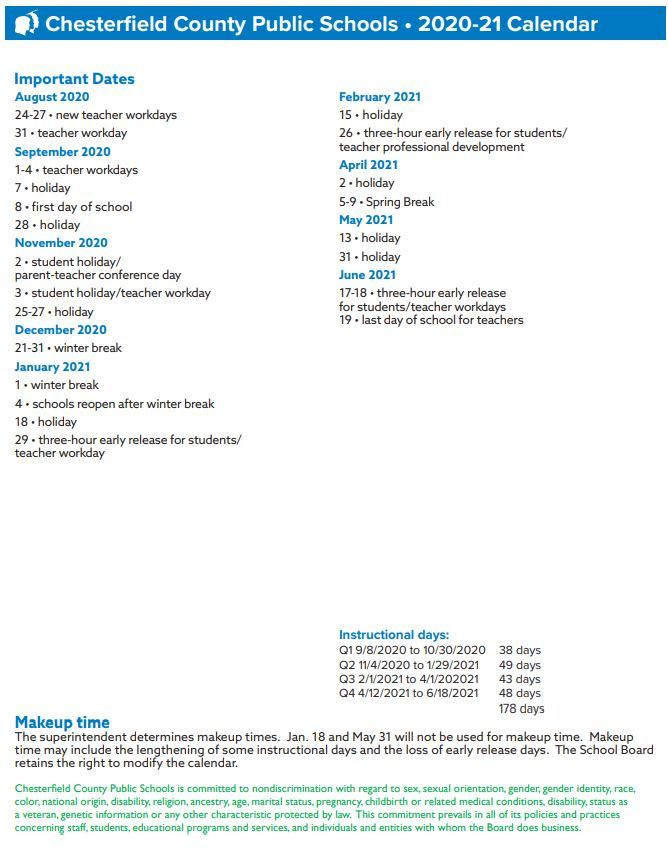table div table+table+table+table+table+table+table+table+table+table+table+table+table+table+table+table+table+table+table+table+table+table+table+table+table+table+table+table+table+table+table+table+table+table+table+table+table+table+table+table+table+table+table+table+table+table+table+table+table div table{width:100%;padding:0}table div table+table+table+table+table+table+table+table+table+table+table+table+table+table+table+table+table+table+table+table+table+table+table+table+table+table+table+table+table+table+table+table+table+table+table+table+table+table+table+table+table+table+table+table+table+table+table+table+table div table img{width:96.23%;padding:0;float:none}table div table+table+table+table+table+table+table+table+table+table+table+table+table+table+table+table+table+table+table+table+table+table+table+table+table+table+table+table+table+table+table+table+table+table+table+table+table+table+table+table+table+table+table+table+table+table+table+table+table div table td{width:100%;padding:0 1.88% 18px}/* styles */# Neighborhood Watch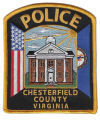Any suspicious activity should always be reported to the local police to investigate.

Bear and fox sightings should be reported to the Animal Control number.

Emergency 911 | Non-emergency 804-748-1251

Animal Control 748-1683

Traffic Hotline 804-318-8084 or speeding@chesterfield.gov

 table div table+table+table+table+table+table+table+table+table+table+table+table+table+table+table+table+table+table+table+table+table+table+table+table+table+table+table+table+table+table+table+table+table+table+table+table+table+table+table+table+table+table+table+table+table+table+table+table+table+table+table+table div table{width:100%;padding:0}table div table+table+table+table+table+table+table+table+table+table+table+table+table+table+table+table+table+table+table+table+table+table+table+table+table+table+table+table+table+table+table+table+table+table+table+table+table+table+table+table+table+table+table+table+table+table+table+table+table+table+table+table div table img{width:96.23%;padding:0;float:none}table div table+table+table+table+table+table+table+table+table+table+table+table+table+table+table+table+table+table+table+table+table+table+table+table+table+table+table+table+table+table+table+table+table+table+table+table+table+table+table+table+table+table+table+table+table+table+table+table+table+table+table+table div table td{width:100%;padding:0 1.88% 18px}/* styles */## Please Serve on our CommitteesWant to participate on a committee? This is a great way to meet and work with neighbors for a common goal: helping our community. We are currently looking for Neighborhood Watch, Covenants and Social Committee chairs.

Please send a message to this newsletter with your interests or contact any Board member, and the BCA will gladly and willingly find a place to use your talents! Many thanks!

 table div table+table+table+table+table+table+table+table+table+table+table+table+table+table+table+table+table+table+table+table+table+table+table+table+table+table+table+table+table+table+table+table+table+table+table+table+table+table+table+table+table+table+table+table+table+table+table+table+table+table+table+table+table+table+table div table{width:100%;padding:0}table div table+table+table+table+table+table+table+table+table+table+table+table+table+table+table+table+table+table+table+table+table+table+table+table+table+table+table+table+table+table+table+table+table+table+table+table+table+table+table+table+table+table+table+table+table+table+table+table+table+table+table+table+table+table+table div table img{width:96.23%;padding:0;float:none}table div table+table+table+table+table+table+table+table+table+table+table+table+table+table+table+table+table+table+table+table+table+table+table+table+table+table+table+table+table+table+table+table+table+table+table+table+table+table+table+table+table+table+table+table+table+table+table+table+table+table+table+table+table+table+table div table td{width:100%;padding:0 1.88% 18px}/* styles */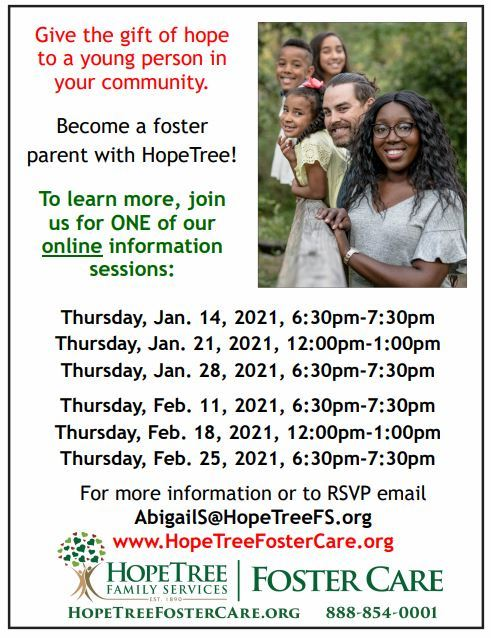table div table+table+table+table+table+table+table+table+table+table+table+table+table+table+table+table+table+table+table+table+table+table+table+table+table+table+table+table+table+table+table+table+table+table+table+table+table+table+table+table+table+table+table+table+table+table+table+table+table+table+table+table+table+table+table+table+table div table{width:100%;padding:0}table div table+table+table+table+table+table+table+table+table+table+table+table+table+table+table+table+table+table+table+table+table+table+table+table+table+table+table+table+table+table+table+table+table+table+table+table+table+table+table+table+table+table+table+table+table+table+table+table+table+table+table+table+table+table+table+table+table div table img{width:96.23%;padding:0;float:none}table div table+table+table+table+table+table+table+table+table+table+table+table+table+table+table+table+table+table+table+table+table+table+table+table+table+table+table+table+table+table+table+table+table+table+table+table+table+table+table+table+table+table+table+table+table+table+table+table+table+table+table+table+table+table+table+table+table div table td{width:100%;padding:0 1.88% 18px}/* styles */## Community Contact List

 /* styles */
 /* styles */ The Birkdale Board Members Ron Buchannan, President 804-740-4212, r_buchanan02@comcast.net * Wayne Bass, Vice- President 639-0795 * Susan Lipp, Secretary /Treasurer 513-978-4708, susanwlipp@yahoo.com * Lee Kemmett, Member-at -Large, 804-739-6405, clkem5@comcast.net ACS West, Community Manager, Lee Ann King 804-282-7451, fax 804-282-9590, E-mail: admin@acswest.org Association Contact Names and Numbers * ARC—Lee Kemmet, 739-6405, clkem5@comcast.net * Covenants Committee--- a volunteer is needed to head this committee- Earl Bishop is currently serving as contact 639-5915, earlbishop8313@gmail.com * Landscaping/Lighting—Wayne Bass, 639-0795; Nick Martello, 739-7487 * Newsletter—Cynthia Steiner, 804-852-3365, newsletter@birkdaleonline.com * Social Committee—VACANT * Neighborhood Watch--VACANT (Won't you please put your name here?) Other Community Contact Names and Numbers * Women’s Club—currently inactive * Swim Team—Joy Blocher, email: collingtonswimteam@gmail.com * Birkdale Golf Course Clubhouse, 739-8800, www.acumengolf.com * Birkdale Golf/Swim Membership, hospitality@acumengolf.com
 table div table+table+table+table+table+table+table+table+table+table+table+table+table+table+table+table+table+table+table+table+table+table+table+table+table+table+table+table+table+table+table+table+table+table+table+table+table+table+table+table+table+table+table+table+table+table+table+table+table+table+table+table+table+table+table+table+table+table+table+table+table div table{width:100%;padding:0}table div table+table+table+table+table+table+table+table+table+table+table+table+table+table+table+table+table+table+table+table+table+table+table+table+table+table+table+table+table+table+table+table+table+table+table+table+table+table+table+table+table+table+table+table+table+table+table+table+table+table+table+table+table+table+table+table+table+table+table+table+table div table img{width:96.23%;padding:0;float:none}table div table+table+table+table+table+table+table+table+table+table+table+table+table+table+table+table+table+table+table+table+table+table+table+table+table+table+table+table+table+table+table+table+table+table+table+table+table+table+table+table+table+table+table+table+table+table+table+table+table+table+table+table+table+table+table+table+table+table+table+table+table div table td{width:100%;padding:0 1.88% 18px}/* styles */